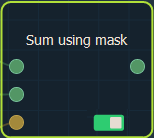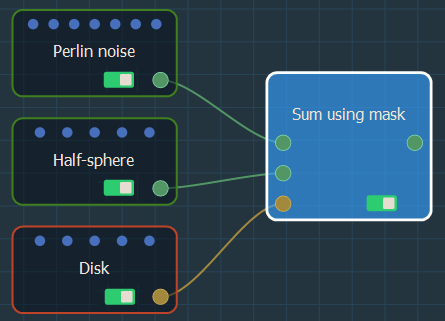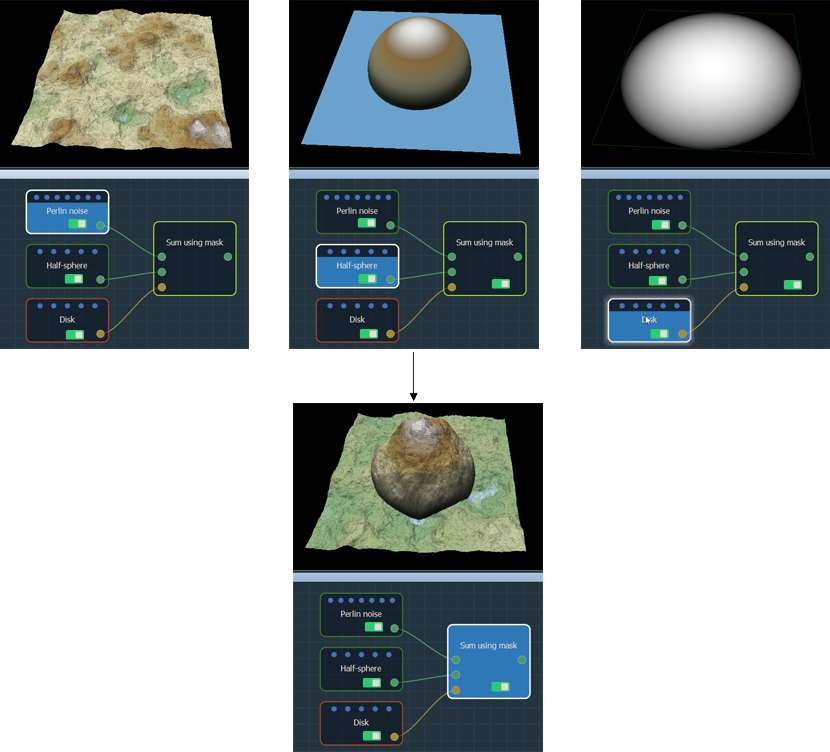This node adds one terrain to another terrain at the locations defined by a mask.

The Sum using mask node works as follows:

• In the white areas, the second terrain is added to the first one.
• In the black areas, only the first terrain is preserved.
• In the gray areas, the second terrain is added to the first terrain, but this time the gray values act as a multiplying coefficient that directly affects this addition.

To add a node, right click in the Graph Editor and select Create Node  > Terrain Composition > Sum using mask.At each vertex:

• If the value of the mask is equal to 1 (white color), the second terrain is added to the first terrain.
• If the value of the mask is equal to 0 (black color), the height of the output terrain is equal to that of the first input terrain. The second input terrain is therefore ignored for this vertex.
• If the value of the mask is between 0 and 1, the height of the second input terrain is multiplied by the value of the mask before being added to the height of the first input terrain.

For example:Both input terrains and the input mask can have different sizes and resolutions. The size and resolution of the output terrain are determined by those of the first terrain and the second terrain and the mask are enlarged or reduced so that their size corresponds exactly to the size of the output terrain.